# The QUANTLIFE Procedure

### Relationship of Quantile Function and Survival Function

Both quantile function and survival function are useful in characterizing a lifetime distribution.

By the definition of the quantile function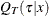,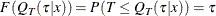In other words, the cumulative distribution function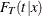mapsto, and thus the corresponding survival function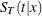mapsto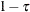.

When you specify the LOG option, the QUANTLIFE procedure fits a linear quantile regression model for a log transformation of the lifetime as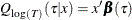where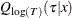is theth quantile of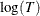at x. The estimated quantile function for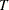given x is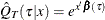, because the quantile function is invariant under a monotone transformation.

You can specify the covariates x in the COVARIATES= data set of the BASELINE statement and the PLOTS=(QUANTILE SURVIVAL) option in the PROC statement. Then the conditional quantile function at x is plotted as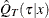against, and the conditional survival function at x is plotted asagainst.